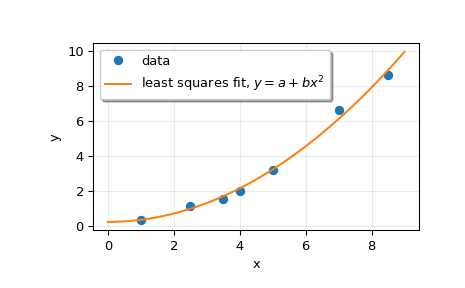# scipy.linalg.lstsq¶

scipy.linalg.lstsq(a, b, cond=None, overwrite_a=False, overwrite_b=False, check_finite=True, lapack_driver=None)[source]

Compute least-squares solution to equation Ax = b.

Compute a vector x such that the 2-norm `|b - A x|` is minimized.

Parameters
a(M, N) array_like

Left-hand side array

b(M,) or (M, K) array_like

Right hand side array

condfloat, optional

Cutoff for ‘small’ singular values; used to determine effective rank of a. Singular values smaller than `rcond * largest_singular_value` are considered zero.

overwrite_abool, optional

Discard data in a (may enhance performance). Default is False.

overwrite_bbool, optional

Discard data in b (may enhance performance). Default is False.

check_finitebool, optional

Whether to check that the input matrices contain only finite numbers. Disabling may give a performance gain, but may result in problems (crashes, non-termination) if the inputs do contain infinities or NaNs.

lapack_driverstr, optional

Which LAPACK driver is used to solve the least-squares problem. Options are `'gelsd'`, `'gelsy'`, `'gelss'`. Default (`'gelsd'`) is a good choice. However, `'gelsy'` can be slightly faster on many problems. `'gelss'` was used historically. It is generally slow but uses less memory.

New in version 0.17.0.

Returns
x(N,) or (N, K) ndarray

Least-squares solution. Return shape matches shape of b.

residues(K,) ndarray or float

Square of the 2-norm for each column in `b - a x`, if `M > N` and `ndim(A) == n` (returns a scalar if b is 1-D). Otherwise a (0,)-shaped array is returned.

rankint

Effective rank of a.

s(min(M, N),) ndarray or None

Singular values of a. The condition number of a is `abs(s / s[-1])`.

Raises
LinAlgError

If computation does not converge.

ValueError

When parameters are not compatible.

`scipy.optimize.nnls`

linear least squares with non-negativity constraint

Notes

When `'gelsy'` is used as a driver, residues is set to a (0,)-shaped array and s is always `None`.

Examples

```>>> from scipy.linalg import lstsq
>>> import matplotlib.pyplot as plt
```

Suppose we have the following data:

```>>> x = np.array([1, 2.5, 3.5, 4, 5, 7, 8.5])
>>> y = np.array([0.3, 1.1, 1.5, 2.0, 3.2, 6.6, 8.6])
```

We want to fit a quadratic polynomial of the form `y = a + b*x**2` to this data. We first form the “design matrix” M, with a constant column of 1s and a column containing `x**2`:

```>>> M = x[:, np.newaxis]**[0, 2]
>>> M
array([[  1.  ,   1.  ],
[  1.  ,   6.25],
[  1.  ,  12.25],
[  1.  ,  16.  ],
[  1.  ,  25.  ],
[  1.  ,  49.  ],
[  1.  ,  72.25]])
```

We want to find the least-squares solution to `M.dot(p) = y`, where `p` is a vector with length 2 that holds the parameters `a` and `b`.

```>>> p, res, rnk, s = lstsq(M, y)
>>> p
array([ 0.20925829,  0.12013861])
```

Plot the data and the fitted curve.

```>>> plt.plot(x, y, 'o', label='data')
>>> xx = np.linspace(0, 9, 101)
>>> yy = p + p*xx**2
>>> plt.plot(xx, yy, label='least squares fit, \$y = a + bx^2\$')
>>> plt.xlabel('x')
>>> plt.ylabel('y')